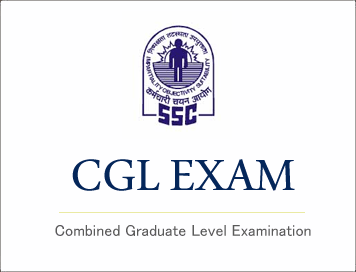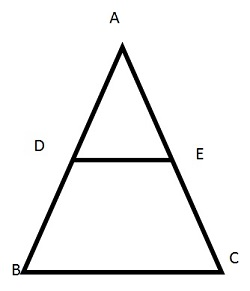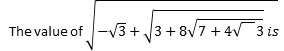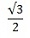# SSC CGL (Tier - 1) Online Exam Paper - 2016 "held on 2 September 2016" Morning Shift (Quantitative Aptitude)## SSC CGL (Tier - 1) Online Exam Paper - 2016 "held on 2 September 2016" Morning Shift (Quantitative Aptitude)

EXAM DATE : 2-September-2016
EXAM START TIME :
10:00:00
EXAM NAME :
SSC Examination 2016

Question 51.If 20 men working 8 hours per day can complete a piece of work in 21 days. How many hours per day must 48 men work to complete the same job in 7 days?

Options:
1) 12
2) 20
3) 10
4) 15

Question 52.Options:
1) 83°
2) 80°
3) 75°
4) 60°

Question 53.A shop keeper earns a profit of 12% on selling a book at 10% discount on the printed price. The ratio of cost price to the printed price of the book is

Options:
1) 45:56
2) 50: 61
3) 99: 125
4) None of these

Question 54.The number of pupils of a class is 55. The ratio of the number of male pupils to the number of female pupils is 5: 6. The number of female pupils is

Options:
1) 11
2) 25
3) 30
4) 35

Question 55.5% more is gained by selling a watch for Rs. 350 than by selling it for Rs. 340. The cost price of the watch is

Options:
1) Rs. 110
2) Rs. 140
3) Rs. 200
4) Rs. 250

Correct Answer: Rs. 200

Question 56.If 60% of the students in a school are boys and number of girls is 812, how many boys are there in the school?

Options:
1) 1128
2) 1218
3) 1821
4) 1281

Question 57.It takes eight hours for a 600 km journey, if 120 km is done by train and the rest by car. It takes 20 minutes more, if 200 km is done by train and the rest by car. The ratio of the speed of the train to that of the car is:

Options:
1) 3:5
2) 3:4
3) 4: 3
4) 4: 5

Question 58.Options:
1) 1
2) -1
3) 0
4) 2

Question 59.If m + n = 1, then the value of m3 + n3 + 3mn is equal to

Options:
1) 0
2) 1
3) 2
4) 3

Question 60.The maximum number of common tangents that can be drawn to two disjoint circles is

Options:
1) 1
2) 2
3) 4
4) Infinitely many

## Study Kit for SSC CGL EXAM

Question 61.In figure, DE || BC. If DE = 3 cm, BC = 6 cm and area of ΔADE = 15 sq cm, then the area of ΔABC isOptions:
1) 75 sq cm
2) 45 sq cm
3) 30 sq cm
4) 60 sq cm

Correct Answer: 60 sq cm

Question 62.If cos4θ - sin4θ = 1/3, then the value of tan2θ is

Options:
1) 1/2
2) 1/3
3) 1/4
4) 1/5

Question 63.If a perfect square, not divisible by 6, be divided by 6, the remainder will be

Options:
1) 1, 3 or 5
2) 1, 2 or 5
3) 1, 3 or 4
4) 1, 2 or 4

Correct Answer: 1, 3 or 4

Question 64.A batsman in his 12th innings makes a score of 120, and thereby increase his average by 5. The average score after 12th innings is

Options:
1) 60
2) 55
3) 65
4) 70

Question 65.Options:
1) 2
2) 4
3) ±2
4) -2

Question 66.Options:
1) 6
2) 12
3) 11
4) 3

Question 67.The side BC of the ΔABC is extended to the point D. If ∠ ACD = 112° and ∠B = 3/4 ∠A, then the value of ∠B is

Options:
1) 64°
2) 48°
3) 46°
4) 50°

Question 68.ΔABC is a right angled triangle, the radius of its circumcircle is 3 cm and the length of its altitude drawn from the opposite vertex to the hypotenuse is 2 cm. Then the area of the triangle is

Options:
1) 12 sq cm
2) 3 sq cm
3) 6 sq cm
4) 5 sq cm

Correct Answer: 6 sq cm

Question 69.The height of a tower is 50√3 m. The angle of elevation of a tower from a distance 50 m from its feet is

Options:
1) 30°
2) 45°
3) 60°
4) 90°

Question 70.The amount of Rs. 10,000 after 2 years, compounded annually with the rate of interest being 10% per annum during the first year and 12% per annum during the second year, would be (in rupees)

Options:
1) 11,320
2) 12,000
3) 12,320
4) 12,500

Question 71.The value of tan80° tan10° + sin270° + sin20° is  tan80° tan10° + sin270° + sin20°

Options:
1) 0
2) 1
3) 2
4Question 72.The bar graph given below shows the per acre yield (in kg) of different countries. Study the graph carefully and answer the questionsThe average yield of the given countries is

Options:
1) 1321/3
2) 1331/3
3) 1341/3
4) 1351/3

Question 73.The bar graph given below shows the per acre yield (in kg) of different countries. Study the graph carefully and answer the questionsBy how much percentage is India's per acre yield more than that of Pakistan's?

Options:
1) 20%
2) 25%
3) 33 1/3%
4) 35%

Question 74.The bar graph given below shows the per acre yield (in kg) of different countries. Study the graph carefully and answer the questionsSrilanka's yield (approximately) is what percentof total yield of all the countries?

Options:
1) 17.8%
2) 16.2%
3) 18.2%
4) 15.4%

Question 75.The bar graph given below shows the per acre yield (in kg) of different countries. Study the graph carefully and answer the questionsWriting the yields of all countries in ascending order, the difference between the sum of yields of first three countries to that of last three countries is

Options:
1) 200 kg
2) 212 kg
3) 172 kg
4) 162 kg

Correct Answer: 172 kg

## Related Posts:

 (Papers) SSC CGL (Tier - 2) Exam - 2017 "held on 21-Feb-2018 " Morning Shift" (Quantitative Ability) (पेपर) एस.एस.सी सीजीएल (टीयर -2) परीक्षा - 2017 "20 फरवरी 2018 को आयोजित" सुबह पाली "(मात्रात्मक क्षमता) (Download) SSC: CGL Tier-II 2013 Exam Paper (Numerical Aptitude) Held On 21.9.2014 (Papers) SSC CGL (Tier - 2) Exam - 2017 "held on 20-Feb-2018 " Morning Shift" (Quantitative Ability) एस.एस.सी. सीजीएल (टियर -1) परीक्षा पेपर 2017 "08 अगस्त 2017" दोपहर की पाली (मात्रात्मक रूझान) SSC CGL (Tier - 1) Online Exam Paper - 2017 "held on 08 August 2017" Afternoon Shift (Quantitative Aptitude)